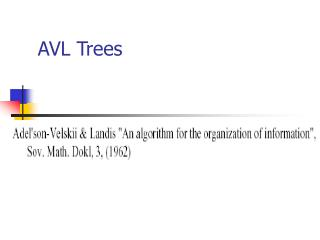DownloadDownload PresentationAVL Trees

# AVL Trees

Télécharger la présentation## AVL Trees

- - - - - - - - - - - - - - - - - - - - - - - - - - - E N D - - - - - - - - - - - - - - - - - - - - - - - - - - -
##### Presentation Transcript

1. AVL Trees

2. AVL Node Structure • The AVL node structure follows the same structure as the binary search tree, with the addition of a term to store the balance factor. Node { int data; Node *left; Node *right; int balanceFactor; }

3. AVL Insert • Now that we have seen how to balance a tree, we are ready to look at the algorithms. • The search and retrieval algorithms are the same as for any binary tree. • However, because the AVL tree is a special case of a binary search tree, you will want to use an inorder traversal method.

4. AVL Insert • As with the BST, all inserts take place at a leaf (or leaf-like) node. • To find the appropriate leaf node, we follow the path from the root, going left when the new data node’s key is less than the root node’s key and right when it’s greater. • Once we have found the leaf, we connect the new node to the leaf and begin to back out of the tree.

5. AVL Insert • It is at this point that the AVL insert differs from the BST insert. • As we back out of the tree, we constantly check the balance of each node. • When we find that a node is out of balance, we balance it and then continue up the tree. • Note that not all inserts will produce an out-of-balance tree.

6. AVL Delete • The delete logic is similar to the BST delete logic. • Again, however, we must make sure that we include the logic to keep the tree balanced.

7. AVL - height 1

8. AVL - height 2

9. AVL - height 3

10. AVL - height 4

11. AVL - height 5

12. AVL - height 6

13. Counting nodes

14. Relationship to Fibonacci • Let N be the fewest number of nodes in an AVL tree of height H • It is straightforward to show thatN = F(H+3) - 1,where F(k) is the kth Fibonacci number • For large values of k,

15. number of nodes • The fewest number of nodes in an AVL tree with height H is given by

16. Solving for H • if we solve this near equality for H, we getH  1.44 log2N • This means that the height of an AVL tree with N nodes is no more than 44% larger than the optimal height of a binary search tree with N nodes